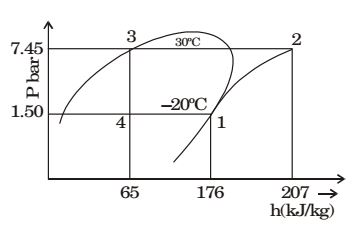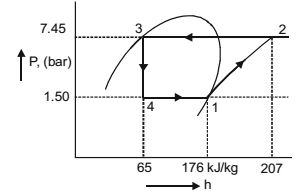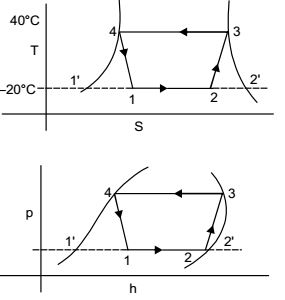## Refrigeration and Air-conditioning Miscellaneous

#### Refrigeration and Air-conditioning

1. A R-12 refrigerant reciprocating compressor operates between the condensing temperature of 30º and evaporator temperature of –20ºC. The clearance volume ratio of the compressor is 0.03. Specific heat ratio of the vapour is 1.15 and the specific volume at the suction is 0.1089 m3/kg. Other properties at various states are given in the figure. To realize 2 tons of refrigeration, the actual volume displacement rate considering the effect of clearance is1. Given: Clearance volume ratio, C = 0.03
Specific volume at suction, υ1 = 0.1089 m3 /kg
Net refrigerating effect = 2 ton = 2 × 3.516 kJ = 7.032 kJ/s
Specific heat ratio, c = 1.15∴ Net refrigerating effect = ṁ(h1 – h4)
or 7.032 = ṁ(176 – 65)
or ṁ = 0.063 kg/s
Volume = 0.063 × 0.1089 = 6.89 × 103 m–3 /s

 Volumetric efficiency = 1 + C - Cp21 / n p1

 Volumetric efficiency = 1 + 0.03 - 0.037.451 / 1.15 1.50

= 0.909
∴ Volume displacement rate considering clearance effect = 6.89 × 10–3 × 0.909 = 6.26 × 10–3 m3 /s

##### Correct Option: A

Given: Clearance volume ratio, C = 0.03
Specific volume at suction, υ1 = 0.1089 m3 /kg
Net refrigerating effect = 2 ton = 2 × 3.516 kJ = 7.032 kJ/s
Specific heat ratio, c = 1.15∴ Net refrigerating effect = ṁ(h1 – h4)
or 7.032 = ṁ(176 – 65)
or ṁ = 0.063 kg/s
Volume = 0.063 × 0.1089 = 6.89 × 103 m–3 /s

 Volumetric efficiency = 1 + C - Cp21 / n p1

 Volumetric efficiency = 1 + 0.03 - 0.037.451 / 1.15 1.50

= 0.909
∴ Volume displacement rate considering clearance effect = 6.89 × 10–3 × 0.909 = 6.26 × 10–3 m3 /s

1. In the window air conditioner, the expansion device used is

1. The capillary tube is used as expansion device

##### Correct Option: A

The capillary tube is used as expansion device

Direction: A refrigerator based on ideal vapour compression cycle operates between the temperature limits of –20°C and 40°C. The refrigerant enters the condenser as saturated vapour and leaves as saturated liquid. The enthalpy and entropy values for saturated liquid and vapour at these temperatures are given in the table below.

 T hf hg sf sg (°C) (kJ/kg) (kJ/kg) (kJ/kgK) (kJ/kgK) –20 20 180 0.07 0.7366 40 80 200 0.3 0.67

1. The COP of the refrigerator is

1.  C.O.P = h2 – h1 h3 – h2

 C.O.P = 164 – 80 = 84 = 2.33 200 – 164 36

##### Correct Option: B

 C.O.P = h2 – h1 h3 – h2

 C.O.P = 164 – 80 = 84 = 2.33 200 – 164 36

1. If refrigerant circulation rate is 0.025 kg/s, the refrigeration effect is equal to

1.h1 = h4 = 80 kJ/kg , s3 = 0.67
h3 = 200 kJ/kg , s2 = 0.67
h1' = 20 kJ/kg , s2' = 0.7366
h2' = 20 kJ/kg , s1' = 0.07
s2 = s1' + x(s2' - s1')
= 0.07 + x + (0.7366 – 0.07)
⇒ 0.67 = 0.07 + 0.6666 x

 ⇒ x = 0.67 - 0.07 = 0.90 0.6666

h2 = h1' + x(h2' - h1')
= 20 + 0.9(180 – 20)
= 20 + 0.9 × 160 = 165 kJ/kg
Refrigerating effect per kg = h2 – h1 = 164 – 80 = 84 kJ/kg
Hence refrigerating effect for 0.025 kg/sec circulation = 84 × 0.025 = 2.1 kW

##### Correct Option: Ah1 = h4 = 80 kJ/kg , s3 = 0.67
h3 = 200 kJ/kg , s2 = 0.67
h1' = 20 kJ/kg , s2' = 0.7366
h2' = 20 kJ/kg , s1' = 0.07
s2 = s1' + x(s2' - s1')
= 0.07 + x + (0.7366 – 0.07)
⇒ 0.67 = 0.07 + 0.6666 x

 ⇒ x = 0.67 - 0.07 = 0.90 0.6666

h2 = h1' + x(h2' - h1')
= 20 + 0.9(180 – 20)
= 20 + 0.9 × 160 = 165 kJ/kg
Refrigerating effect per kg = h2 – h1 = 164 – 80 = 84 kJ/kg
Hence refrigerating effect for 0.025 kg/sec circulation = 84 × 0.025 = 2.1 kW

1. In a vapour compression refrigeration system, liquids to suction heat exchanger is used to

1. NA

NA